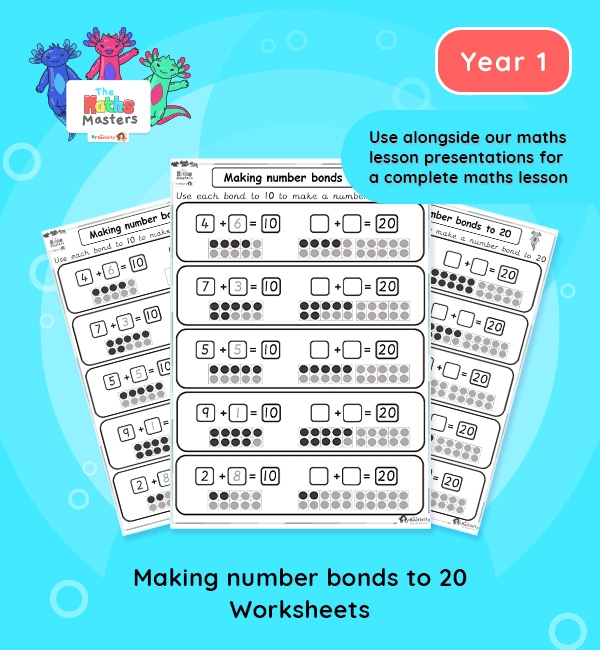# Year 1 | Making Number Bonds to 20 Worksheets## Year 1 addition and subtraction worksheets

Aligned with the maths mastery approach, these Year 1 | Making Number Bonds to 20 Worksheets are designed to be used alongside our Year 1 | Making Number Bonds to 20 Lesson Presentation Lesson Presentation for a complete maths lesson. The maths worksheets requires children to use their knowledge of number bonds to 10  and ten frames to figure out number bonds to 20. The strips can also be cut up and used individually in children’s books to demonstrate knowledge and progression, without the need for a whole worksheet.

Small Steps: Find and make number bonds.

NC Links: Represent and use number bonds and related subtraction facts within 20. * Read, write and interpret mathematical statements involving addition, subtraction and equals signs. *Add and subtract one-digit and two-digit numbers to 20, including zero.

TAF Statements: Working towards: Add and subtract (one digit numbers) explaining their method verbally, in pictures, or using apparatus. Recall at least four of the six number bonds for 10 and reason about associated facts.

Working At: Recall all the number bonds to and within 10 and use these to reason with and calculate bonds to and within 20, recognising other associated additive relationships.

Greater Depth – Use reasoning about number and relationships to solve more complex problems and explain their thinking.

Ready-to-progress criteria:  1NF-1 Develop fluency in addition and subtraction facts within 10

Future applications: Add and subtract across 10.

1AS-2 Read, write and interpret equations containing addition(+), subtraction (-), and equals (=) symbols, and relate additive expressions and equations to real-life contexts.

## Recently Viewed